Anúncio

# Verbal Tenses

31 de Jan de 2023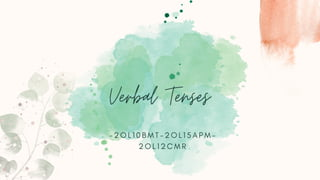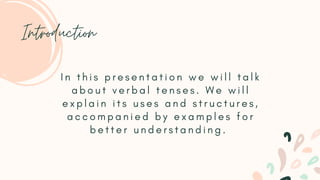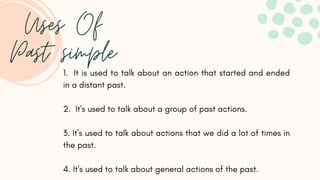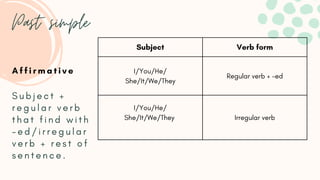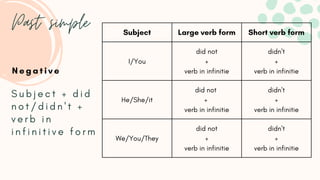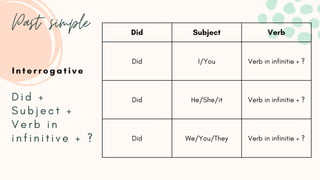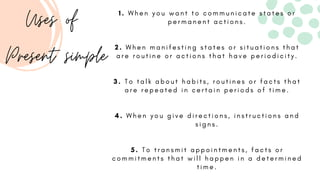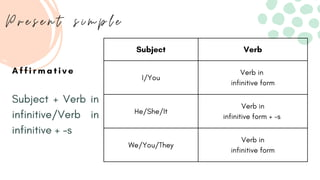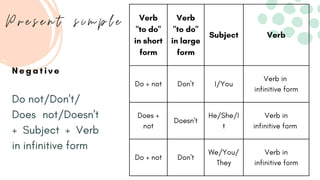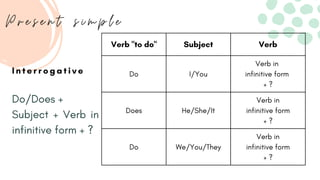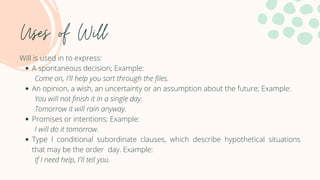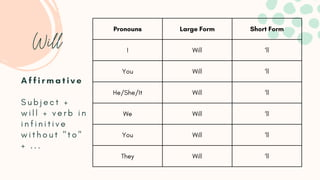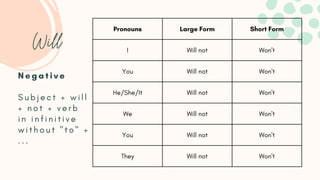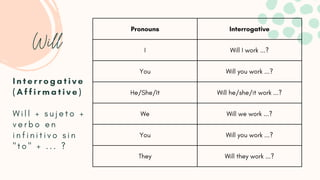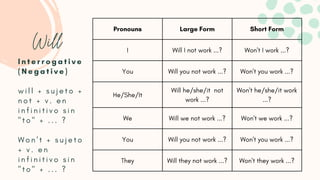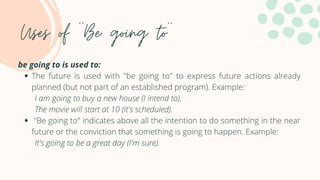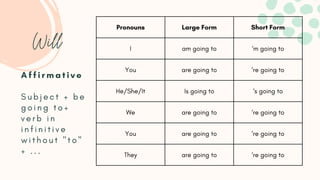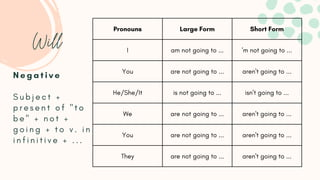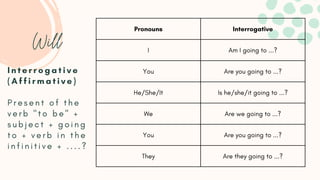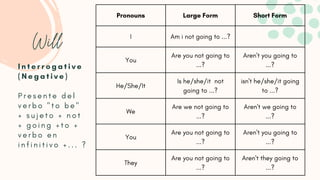1 de 26
Anúncio

### Verbal Tenses

1. Verbal Tenses - 2 O L 1 0 B M T - 2 O L 1 5 A P M - 2 O L 1 2 C M R
2. Contents 1 . P a s t s i m p l e . 2 . P r e s e n t s i m p l e . 3 . F u t u r e s i m p l e .
3. Introduction I n t h i s p r e s e n t a t i o n w e w i l l t a l k a b o u t v e r b a l t e n s e s . W e w i l l e x p l a i n i t s u s e s a n d s t r u c t u r e s , a c c o m p a n i e d b y e x a m p l e s f o r b e t t e r u n d e r s t a n d i n g .
4. About us B e a t r i z M e j í a s C a r l o s M o n t e r o A n d r é s P r a d a s
5. Past simple
6. Uses Of Past simple 1. It is used to talk about an action that started and ended in a distant past. 2. It's used to talk about a group of past actions. 3. It's used to talk about actions that we did a lot of times in the past. 4. It's used to talk about general actions of the past.
7. Past simple A f f i r m a t i v e S u b j e c t + r e g u l a r v e r b t h a t f i n d w i t h - e d / i r r e g u l a r v e r b + r e s t o f s e n t e n c e . Subject Verb form I/You/He/ She/It/We/They Regular verb + -ed I/You/He/ She/It/We/They Irregular verb
8. Past simple N e g a t i v e S u b j e c t + d i d n o t / d i d n ' t + v e r b i n i n f i n i t i v e f o r m Subject Large verb form Short verb form I/You did not + verb in infinitie didn't + verb in infinitie He/She/it did not + verb in infinitie didn't + verb in infinitie We/You/They did not + verb in infinitie didn't + verb in infinitie
9. Past simple I n t e r r o g a t i v e D i d + S u b j e c t + V e r b i n i n f i n i t i v e + ? Did Subject Verb Did I/You Verb in infinitie + ? Did He/She/it Verb in infinitie + ? Did We/You/They Verb in infinitie + ?
10. Present Simple
11. 1 . W h e n y o u w a n t t o c o m m u n i c a t e s t a t e s o r p e r m a n e n t a c t i o n s . 2 . W h e n m a n i f e s t i n g s t a t e s o r s i t u a t i o n s t h a t a r e r o u t i n e o r a c t i o n s t h a t h a v e p e r i o d i c i t y . 3 . T o t a l k a b o u t h a b i t s , r o u t i n e s o r f a c t s t h a t a r e r e p e a t e d i n c e r t a i n p e r i o d s o f t i m e . 4 . W h e n y o u g i v e d i r e c t i o n s , i n s t r u c t i o n s a n d s i g n s . 5 . T o t r a n s m i t a p p o i n t m e n t s , f a c t s o r c o m m i t m e n t s t h a t w i l l h a p p e n i n a d e t e r m i n e d t i m e . Uses of Present simple
12. Subject Verb I/You Verb in infinitive form He/She/It Verb in infinitive form + -s We/You/They Verb in infinitive form A f f i r m a t i v e P r e s e n t s i m p l e Subject + Verb in infinitive/Verb in infinitive + -s
13. N e g a t i v e P r e s e n t s i m p l e Verb "to do" in short form Verb "to do" in large form Subject Verb Do + not Don't I/You Verb in infinitive form Does + not Doesn't He/She/I t Verb in infinitive form Do + not Don't We/You/ They Verb in infinitive form Do not/Don't/ Does not/Doesn't + Subject + Verb in infinitive form
14. I n t e r r o g a t i v e P r e s e n t s i m p l e Verb "to do" Subject Verb Do I/You Verb in infinitive form + ? Does He/She/It Verb in infinitive form + ? Do We/You/They Verb in infinitive form + ? Do/Does + Subject + Verb in infinitive form + ?
15. Future Simple
16. Uses of Will A spontaneous decision; Example: An opinion, a wish, an uncertainty or an assumption about the future; Example: Promises or intentions; Example: Type I conditional subordinate clauses, which describe hypothetical situations that may be the order day. Example: Will is used in to express: Come on, I'll help you sort through the files. You will not finish it in a single day. Tomorrow it will rain anyway. I will do it tomorrow. If I need help, I'll tell you.
17. Pronouns Large Form Short Form I Will ‘ll You Will ‘ll He/She/It Will ‘ll We Will ‘ll You Will ‘ll They Will ‘ll Will A f f i r m a t i v e S u b j e c t + w i l l + v e r b i n i n f i n i t i v e w i t h o u t " t o " + . . .
18. Pronouns Large Form Short Form I Will not Won't You Will not Won't He/She/It Will not Won't We Will not Won't You Will not Won't They Will not Won't Will N e g a t i v e S u b j e c t + w i l l + n o t + v e r b i n i n f i n i t i v e w i t h o u t " t o " + . . .
19. Pronouns Interrogative I Will I work ...? You Will you work ...? He/She/It Will he/she/it work ...? We Will we work ...? You Will you work ...? They Will they work ...? Will I n t e r r o g a t i v e ( A f f i r m a t i v e ) W i l l + s u j e t o + v e r b o e n i n f i n i t i v o s i n " t o " + . . . ?
20. Pronouns Large Form Short Form I Will I not work ...? Won't I work ...? You Will you not work ...? Won't you work ...? He/She/It Will he/she/it not work ...? Won't he/she/it work ...? We Will we not work ...? Won't we work ...? You Will you not work ...? Won't you work ...? They Will they not work ...? Won't they work ...? Will I n t e r r o g a t i v e ( N e g a t i v e ) w i l l + s u j e t o + n o t + v . e n i n f i n i t i v o s i n " t o " + . . . ? W o n ' t + s u j e t o + v . e n i n f i n i t i v o s i n " t o " + . . . ?
21. Uses of ''Be going to'' The future is used with "be going to" to express future actions already planned (but not part of an established program). Example: "Be going to" indicates above all the intention to do something in the near future or the conviction that something is going to happen. Example: be going to is used to: I am going to buy a new house (I intend to). The movie will start at 10 (it's scheduled). It's going to be a great day (I'm sure).
22. Pronouns Large Form Short Form I am going to ‘m going to You are going to ‘re going to He/She/It Is going to ‘s going to We are going to ‘re going to You are going to ‘re going to They are going to ‘re going to Will A f f i r m a t i v e S u b j e c t + b e g o i n g t o + v e r b i n i n f i n i t i v e w i t h o u t " t o " + . . .
23. Pronouns Large Form Short Form I am not going to ... 'm not going to ... You are not going to ... aren't going to ... He/She/It is not going to ... isn't going to ... We are not going to ... aren't going to ... You are not going to ... aren't going to ... They are not going to ... aren't going to ... Will N e g a t i v e S u b j e c t + p r e s e n t o f " t o b e " + n o t + g o i n g + t o v . i n i n f i n i t i v e + . . .
24. Pronouns Interrogative I Am I going to ...? You Are you going to ...? He/She/It Is he/she/it going to ...? We Are we going to ...? You Are you going to ...? They Are they going to ...? Will I n t e r r o g a t i v e ( A f f i r m a t i v e ) P r e s e n t o f t h e v e r b " t o b e " + s u b j e c t + g o i n g t o + v e r b i n t h e i n f i n i t i v e + . . . . ?
25. Pronouns Large Form Short Form I Am i not going to ...? You Are you not going to ...? Aren't you going to ...? He/She/It Is he/she/it not going to ...? isn't he/she/it going to ...? We Are we not going to ...? Aren't we going to ...? You Are you not going to ...? Aren't you going to ...? They Are you not going to ...? Aren't they going to ...? Will I n t e r r o g a t i v e ( N e g a t i v e ) P r e s e n t e d e l v e r b o " t o b e " + s u j e t o + n o t + g o i n g + t o + v e r b o e n i n f i n i t i v o + . . . ?
26. THE END
Anúncio